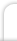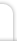Mathematics
 Author: William Pantoja Illustrations: William Pantoja Date: January 14, 2012

Contents

1. Overview

2. Numbers

2.1. Decimal Numbers

2.1.1. Basic Numbers

Number Name Amount
0 zero
1 one
2 two ••
3 three •••
4 four ••••
5 five •••••
6 six ••••• •
7 seven ••••• ••
8 eight ••••• •••
9 nine ••••• ••••
10 ten ••••• •••••

2.1.2. The "Teens"

Number Name Amount
11 eleven ••••• ••••• •
12 twelve ••••• ••••• ••
13 thirteen ••••• ••••• •••
14 fourteen ••••• ••••• ••••
15 fifteen ••••• ••••• •••••
16 sixteen ••••• ••••• ••••• •
17 seventeen ••••• ••••• ••••• ••
18 eighteen ••••• ••••• ••••• •••
19 nineteen ••••• ••••• ••••• ••••

2.1.3. The "Tens"

Number Name Amount
20 twenty two tens
30 thirty three tens
40 fourty four tens
50 fifty five tens
60 sixty six tens
70 seventy seven tens
80 eighty eight tens
90 ninety nine tens

2.1.4. Larger Numbers

A comma is used with larger numbers to group digits in sets of three. After one million some of these definitions differ depending on which country you live in. The definitions below are used in the United States and France.

Number Name Amount
100 one hundred ten tens
1,000 one thousand ten one hundreds
10,000 ten thousand ten one thousands
100,000 one hundred thousand one hundred one thousands
1,000,000 one million one thousand one thousands
1,000,000,000 one billion one thousand one millons
1,000,000,000,000 one trillion one thousand one billions
1,000,000,000,000,000 one quadrillion one thousand one trillions
1,000,000,000,000,000,000 one quintillion one thousand one quadrillions
1,000,000,000,000,000,000,000 one sextillion one thousand one quintillions
1,000,000,000,000,000,000,000,000 one septillion one thousand one sextillions
1,000,000,000,000,000,000,000,000,000 one octillion one thousand one septillions
1 followed by 100 zeros one googol
1 followed by a googol zeros one googolplex

2.1.5. Fractions

Digits to the right of the decimal point represent the fractional value of a number. Each decimal place is one tenth the magnitude of the decimal place to its immediate left. When writing our pronouncing a decimal number greater than one, the decimal point is written and prounounced as "and".

Number Name Fraction
0.1 one tenth 1/10
0.01 one hundredth 1/100
0.001 one thousandth 1/1000
0.0001 one ten thousandth 1/10000
0.00001 one hundred thousandth 1/100000

Examples:

0.27

is pronounced twenty-seven hundredths.

329.582

is pronounced three hundred twenty-nine and five hundred eighty-two thousandths.

\$7,293.23

is pronounced seven thousand two hundred ninety-three and twenty-three dollars.

2.1.6. Roman Numerals

 • Roman numerals has no representation of zero. • The numbers are created starting from the largest digit on the left (skipping any zeros) to the smallest on the right. • If a numeral to the immediate left of a numeral is smaller, the numeral to the left is subtracted from the numeral on the right (e.g., IX = 10 - 1 = 9).
Number Value
I 1
II 2
III 3
IV 4
V 5
VI 6
VII 7
VIII 8
IX 9
X 10
XL 40
L 50
XC 90
C 100
CD 400
D 500
CM 900
M 1,000
MV 4,000
V 5,000
MX 9,000
X 10,000
XL 40,000
L 50,000
XC 90,000
C 100,000
CD 400,000
D 500,000
CM 900,000
M 1,000,000

Examples:

LXXXVII

is 87.

MMCDXCIII

is 2,493.

DCMMXXXXL

is 602,350.

2.1.7. Other Bases

Numbers may be represented in other bases than 10. A single digit in a number is a value between 0 and one less then the base inclusive (e.g., base 8 uses the digits 0 through 7). Some bases, such as base sixteen (or hexadecimal) use alpha characters to represent digits greater than nine. A number written in a base other than base 10 (decimal) will have its base written in subscript after the number (e.g., 2538 is a base eight number).

Decimal (base-10) Binary (base-2) Octal (base-8) Hexadecimal (base-16)
0 02 08 016
1 12 18 116
2 102 28 216
3 112 38 316
4 1002 48 416
5 1012 58 516
6 1102 68 616
7 1112 78 716
8 10002 108 816
9 10012 118 916
10 10102 128 A16
11 10112 138 B16
12 11002 148 C16
13 11012 158 D16
14 11102 168 E16
15 11112 178 F16
16 100002 208 1016
17 100012 218 1116
18 100102 228 1216
19 100112 238 1316
20 101002 248 1416
21 101012 258 1516
22 101102 268 1616
23 101112 278 1716
24 110002 308 1816

3. Algebra

Identity

a + 0 = a

Inverse

a + (-a) = 0

Associativity

(a + b) + c = a + (b + c)

Commutativity

a + b = b + a

3.2. Subtraction

Identity

a - 0 = a

Inverse

a - a = 0

Associativity

(a - b) - c = a - (b - c)

Commutativity

Subtraction has no commutativity.

a - bb - a

3.3. Multiplication

Identity

a × 1 = a

Inverse

a ÷ a = 1

Associativity

(a × b) × c = a × (b × c)

Commutativity

a × b = b × a

3.4. Division

Identity

a ÷ 1 = a

Inverse

a × a = 1

Associativity

(a ÷ b) ÷ c = a ÷ (b ÷ c)

Commutativity

Division has no commutativity.

a ÷ bb ÷ a

3.5. Exponentiation

an = a × a × a × a × … × a (n number of times)

Identity

an × am = an + m

(an)m = an × m

(a × b)n = an × bn

Inverse

alogab = b

elna = a

Associativity

Exponentiation has no associativity.

abc ≠ (ab)c

Commutativity

Exponentiation has no commutativity.

abba

Properties

Exponentiation has these properties.

a0 = 1

Note that in the case of 00 the value may differ depending on the application. In general, 00 = 1.

a1/n = na

an/m = man